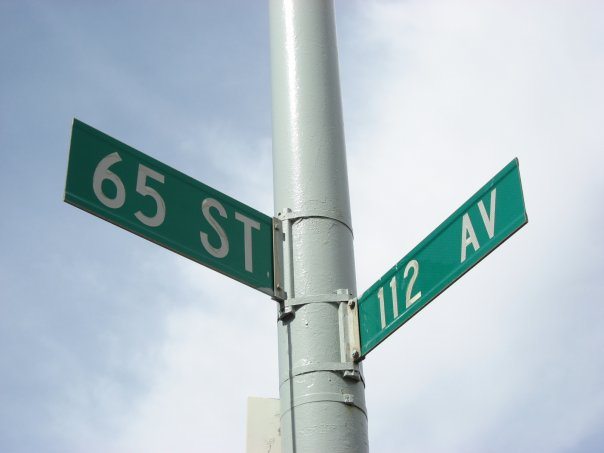## Quality of LivingCities in Alberta tend to be laid out as rectangular grids of blocks. Blocks are labeled with coordinates 0 to R−1 from north to south and 0 to C−1 from west to east.

The quality of living in each particular block has been ranked by a distinct number, called quality rank, between 1 and R*C, where 1 is the best and R*C is the worst.

The city planning department wishes to identify a rectangular set of blocks with dimensions H from north to south and W from west to east, such that the median quality rank among all blocks in the rectangle is the best.

H and W are odd numbers not exceeding R and C respectively. The median quality rank among an odd number of quality ranks is defined to be the quality rank m in the set such that the number of quality ranks better than m equals the number of quality ranks worse than m.

### Input Format

The first line of input will contain the four integers R C H W, where R and C represent the total size of the city, and H and W represent the dimensions of the set of blocks.

The next R lines each contain C integers, denoting an array Q where Q[a][b] is the quality rank for the block labeled a from north to south and b from west to east.

### Output Format

A single integer, the best (numerically smallest) possible median quality rank of an H by W rectangle of blocks.

### Sample Input 1

```5 5 3 3
5 11 12 16 25
17 18 2 7 10
4 23 20 3 1
24 21 19 14 9
6 22 8 13 15```

`9`

### Explanation

```R=5, C=5, H=3, W=3,
Q=  5 11 12 16 25
17 18  2  7 10
4 23 20  3  1
24 21 19 14  9
6 22  8 13 15```

For this example, the best (numerically smallest) median quality rank of 9 is achieved by the middle-right rectangle of Q shown in bold.

### Sample Input 2

```2 6 1 5
6 1 2 11 7 5
9 3 4 10 12 8```

### Sample Output 2

`5`

Subtask 1 [20% of points]: Assume R and C do not exceed 30.
Subtask 2 [20% of points]: Assume R and C do not exceed 100.
Subtask 3 [20% of points]: Assume R and C do not exceed 300.
Subtask 4 [20% of points]: Assume R and C do not exceed 1 000.
Subtask 5 [20% of points]: Assume R and C do not exceed 3 000.

Point Value: 15 (partial)
Time Limit: 10.00s
Memory Limit: 256M